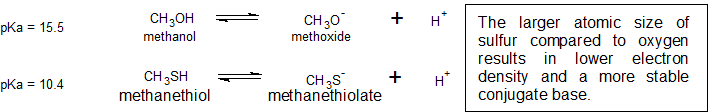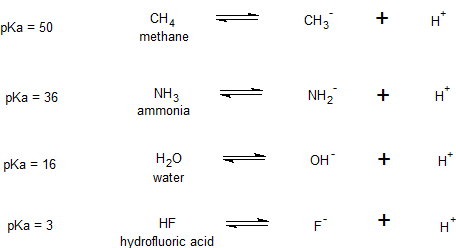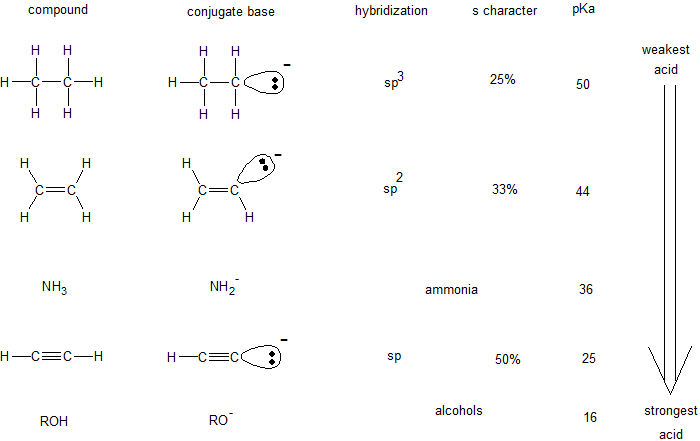# 1.15: Predicting Relative Acidity

Learning Objective

• Predict relative strengths of acids and bases from their structure, bonding and resonance

Since compounds are neutral, it can be difficult to evaluate and compare their overall stability without going through the tedious process of performing bond energy calculations.

When acidic compounds donate hydrogen ions or accepts electrons, they become ionized. It is much easier to compare ions because we can evaluate the charge density. The lower the charge density, the more stable the ion. Conversely, the higher the charge density, the less stable the ion. Charge density is analogous to density of matter. We place charge in the numerator, instead of mass, and volume can still be found in the denominator.

$\text{charge density} =\dfrac{\text{charge}}{\text{volume}}$

The six strong acids (HCl, HBr, HI, HNO3, H2SO4, HClO4) fully ionize to form the highly stable anions (Cl-, Br-, I-, NO3-, SO42-, ClO4-) respectively.

For the remaining weak acids (HA), we can determine their relative acidity by comparing the relative electron densities of their conjugate bases (A-).

the lower the electron density, the more stable the conjugate base

Structural Effects on Electron Density - Four Considerations

There are four main considerations for evaluating electron density.

1. Identity of the element or atoms holding the charge
2. Can the charge be delocalized by resonance?
3. Are there any inductive effects?
4. Hybridization of orbital holding the charge

These considerations are listed in order of importance and are explained individually, but must be looked at collectively.

Identity of the Element

When comparing the identity of the elements, it depends on the positional relationship of the elements on the periodic table.

Within a Group (aka down a column) As we move down the periodic table, the electrons are occupying higher energy subshells creating a larger atomic size and volume. As the volume increases, the electron density decreases.

Figure $$\PageIndex{1}$$ shows spheres representing the atoms of the s and p blocks from the periodic table to scale, showing the two trends for the atomic radius.Figure $$\PageIndex{1}$$: Atomic Radii Trends on the Periodic Table. Although there are some reversals in the trend (e.g., see Po in the bottom row), atoms generally get smaller as you go across the periodic table and larger as you go down any one column. Numbers are the radii in pm.

This relationship of atomic size and electron density is illustrated when we compare the relative acidities of methanol, CH3OH, with methanethiol, CH3SH. The lower pKa value of 10.4 for methanethiol indicates that it is a stronger acid than methanol with a pKa value of 15.5. It is important to remember that neither compound is considered an acid. These relationships become useful when trying to deprotonate compounds to increase their chemical reactivity in non-aqueous reaction conditions.Across a Period (aka across a row) As we move across a period of the main group elements, the valence electrons all occupy orbitals in the same shell. These electrons have comparable energy, so this factor does not help us discern differences relative stability. Differences in electronegativity are now the dominant factor. This trend is shown when comparing the pKa values of methane, ammonia, water, and hydrofluoric acid reflects the relative electonegativities of the C < N < O < F.## Periodic trends

First, we will focus on individual atoms, and think about trends associated with the position of an element on the periodic table. We’ll use as our first models the simple organic compounds ethane, methylamine, and ethanol, but the concepts apply equally to more complex biomolecules, such as the side chains of alanine, lysine, and serine.Figure $$\PageIndex{2}$$: Horizontal periodic trend in acidity and basicity. (CC-NC-SA; Timothy Soderberg via UMn Morris Digital Well)

We can see a clear trend in acidity as we move from left to right along the second row of the periodic table from carbon to nitrogen to oxygen. The key to understanding this trend is to consider the hypothetical conjugate base in each case: the more stable (weaker) the conjugate base, the stronger the acid. Look at where the negative charge ends up in each conjugate base. In the ethyl anion, the negative charge is borne by carbon, while in the methylamine anion and ethoxide anion the charges are located on a nitrogen and an oxygen, respectively. Remember the periodic trend in electronegativity (section 2.3A): it also increases as we move from left to right along a row, meaning that oxygen is the most electronegative of the three, and carbon the least. The more electronegative an atom, the better it is able to bear a negative charge. Thus, the ethoxide anion is the most stable (lowest energy, least basic) of the three conjugate bases, and the ethyl anion is the least stable (highest energy, most basic).

We can use the same set of ideas to explain the difference in basicity between water and ammonia.By looking at the pKavalues for the appropriate conjugate acids, we know that ammonia is more basic than water. Oxygen, as the more electronegative element, holds more tightly to its lone pair than the nitrogen. The nitrogen lone pair, therefore, is more likely to break away and form a new bond to a proton - it is, in other words, more basic. Once again, a more reactive (stronger) conjugate base means a less reactive (weaker) conjugate acid.

When moving vertically within a given column of the periodic table, we again observe a clear periodic trend in acidity. This is best illustrated with the halides: basicity, like electronegativity, increases as we move up the column.Figure $$\PageIndex{4}$$: Vertical periodic trend in acidity and basicity. (CC-NC-SA; Timothy Soderberg via UMn Morris Digital Well)

Conversely, acidity in the haloacids increases as we move down the column.

In order to make sense of this trend, we will once again consider the stability of the conjugate bases. Because fluorine is the most electronegative halogen element, we might expect fluoride to also be the least basic halogen ion. But in fact, it is the least stable, and the most basic! It turns out that when moving vertically in the periodic table, the size of the atom trumps its electronegativity with regard to basicity. The atomic radius of iodine is approximately twice that of fluorine, so in an iodine ion, the negative charge is spread out over a significantly larger volume:Figure $$\PageIndex{5}$$: In conjugate bases the size of the ion controls basicity. ()

This illustrates a fundamental concept in organic chemistry that is important enough to put in red:

Electrostatic charges, whether positive or negative, are more stable when they are ‘spread out’ than when they are confined to one atom.

We will see this idea expressed again and again throughout our study of organic reactivity, in many different contexts. For now, the concept is applied only to the influence of atomic radius on anion stability. Because fluoride is the least stable (most basic) of the halide conjugate bases, HF is the least acidic of the haloacids, only slightly stronger than acetic acid. HI, with a pKa of about -9, is one the strongest acids known.

More importantly to the study of biological organic chemistry, this trend tells us that thiols are more acidic than alcohols. The pKa of the thiol group on the cysteine side chain, for example, is approximately 8.3, while the pKa for the hydroxyl on the serine side chain is on the order of 17.

To reiterate: acid strength increases as we move to the right along a row of the periodic table, and as we move down a column.Example $$\PageIndex{1}$$:

Draw the structure of the conjugate base that would form if the compound below were to react with 1 molar equivalent of sodium hydroxide:Solution

Is resonance possible to localize the charge?

In the previous section we focused our attention on periodic trends - the differences in acidity and basicity between groups where the exchangeable proton was bound to different elements. Now, it is time to think about how the structure of different organic groups contributes to their relative acidity or basicity, even when we are talking about the same element acting as the proton donor/acceptor. When evaluating conjugate bases for the presence of the resonance contributors, remember to look for movable electrons as described in section 1.10 of this chapter. Delocalizing electrons over two or more atoms lowers the electron density.

The first model pair we will consider is ethanol and acetic acid, but the conclusions we reach will be equally valid for all alcohol and carboxylic acid groups. Despite the fact that they are both oxygen acids, the pKa values of ethanol and acetic acid are very different. What makes a carboxylic acid so much more acidic than an alcohol? As before, we begin by considering the conjugate bases.Figure $$\PageIndex{8}$$:  (CC-NC-SA; Timothy Soderberg via UMn Morris Digital Well)

In both species, the negative charge on the conjugate base is held by an oxygen, so periodic trends cannot be invoked. For acetic acid, however, there is a key difference: a resonance contributor can be drawn in which the negative charge is localized on the second oxygen of the group. The two resonance forms for the conjugate base are equal in energy, according to our ‘rules of resonance’ (section 2.2C). What this means, you may recall, is that the negative charge on the acetate ion is not located on one oxygen or the other: rather it is shared between the two. Chemists use the term ‘delocalization of charge’ to describe this situation. In the ethoxide ion, by contrast, the negative charge is ‘locked’ on the single oxygen – it has nowhere else to go.

Now is the time to think back to that statement from the previous section that was so important that it got printed in bold font in its own paragraph – in fact, it is so important that we’ll just say it again: "Electrostatic charges, whether positive or negative, are more stable when they are ‘spread out’ than when they are confined to one atom." Now, we are seeing this concept in another context, where a charge is being ‘spread out’ (in other words, delocalized) by resonance, rather than simply by the size of the atom involved.

The delocalization of charge by resonance has a very powerful effect on the reactivity of organic molecules, enough to account for the difference of over 12 pKa units between ethanol and acetic acid (and remember, pKa is a log expression, so we are talking about a difference of over 1012 between the acidity constants for the two molecules). The acetate ion is that much more stable than the ethoxide ion, all due to the effects of resonance delocalization.

The resonance effect also nicely explains why a nitrogen atom is basic when it is in an amine, but not basic when it is part of an amide group. Recall that in an amide, there is significant double-bond character to the carbon-nitrogen bond, due to a second resonance contributor in which the nitrogen lone pair is part of a p bond.Figure $$\PageIndex{9}$$:  (CC-NC-SA; Timothy Soderberg via UMn Morris Digital Well)

While the electron lone pair of an amine nitrogen is ‘stuck’ in one place, the lone pair on an amide nitrogen is delocalized by resonance. Notice that in this case, we are extending our central statement to say that electron density – in the form of a lone pair – is stabilized by resonance delocalization, even though there is not a negative charge involved. Here’s another way to think about it: the lone pair on an amide nitrogen is not available for bonding with a proton – these two electrons are too ‘comfortable’ being part of the delocalized pi-bonding system. The lone pair on an amine nitrogen, by contrast, is not part of a delocalized p system, and is very ready to form a bond with any acidic proton that might be nearby.

Often it requires some careful thought to predict the most acidic proton on a molecule. Ascorbic acid, also known as Vitamin C, has a pKa of 4.1.There are four hydroxyl groups on this molecule – which one is the most acidic? If we consider all four possible conjugate bases, we find that there is only one for which we can delocalized the negative charge over two oxygen atoms.

Example $$\PageIndex{1}$$:

Rank the compounds below from most acidic to least acidic, and explain your reasoning.(CC-NC-SA; Timothy Soderberg via UMn Morris Digital Well)

### Inductive Effects

The inductive effect is an experimentally observed effect of the transmission of charge through a chain of atoms in a molecule, resulting in a permanent dipole in a bond. Inductive effects decrease quickly with distance. The inductive effect can be electron donating which helps stabilize positive charge. Alkyl groups (hydrocarbons) are inductive electron donators. The inductive effect can also be electron withdrawing. Electronegativity indicates the strength of electron withdrawing induction. Halogens are inductive electron withdrawing groups.

The effects of induction on relative acidity can also be seen when comparing acetic acid with trifluoroacetic acid. The difference in acidity does not have to do with resonance delocalization because no additional resonance structures can be drawn for the fluorinated molecule. The fluorine atoms inductively pull some of the electron density away from the carboxylate ion to further delocalize the negative charge of the conjugate base.Compare the pKa values of acetic acid and its mono-, di-, and tri-chlorinated derivatives:(CC-NC-SA; Timothy Soderberg via UMn Morris Digital Well)

The presence of the chlorines clearly increases the acidity of the carboxylic acid group. A chlorine atom is more electronegative than a hydrogen, and thus is able to ‘induce’, or ‘pull’ electron density towards itself, away from the carboxylate group. In helping to further spread out the electron density of the conjugate base, which as we know has a stabilizing effect. In this context, the chlorine substituent is called an electron-withdrawing group. Notice that the pKa-lowering effect of each chlorine atom, while significant, is not as dramatic as the delocalizing resonance effect illustrated by the difference in pKa values between an alcohol and a carboxylic acid. In general, resonance effects are more powerful than inductive effects. The inductive electron-withdrawing effect of the chlorines takes place through covalent bonds, and its influence decreases markedly with distance – thus a chlorine two carbons away from a carboxylic acid group has a decreased effect compared to a chlorine just one carbon away.

Exercise

Rank the compounds below from most acidic to least acidic, and explain your reasoning.Solution

Orbital Hybridization

The hybridization of an orbital affects its electronegativity. Within a shell, the s orbitals occupy the region closer to the nucleus than the p orbitals. Therefore, the spherical s orbitals are more electronegative than the lobed p orbitals. The relative electronegativity of hybridized orbitals is sp > sp2 > sp3. This trend indicates the sp hybridized orbitals are more stable with a -1 charge than sp3 hybridized orbitals. The table below shows how orbital hybridization compares with the identity of the atom when predicting relative acidity.Guided Practice

Let's practice by comparing the relative acidity of phenol with acetic acid.Which compound is the stronger acid - phenol or acetic acid?

To answer this question, we draw all the relevant resonance contributors for each conjugate base, phenoxide and acetate, respectively.

Phenoxide has four resonance contributors, but three of the contributors have a negative charge on a carbon atom while both resonance contributors for acetate have a negative charge on the more electronegative element oxygen. There are no inductive effects or orbital hybridization differences to consider in this example, so we would predict acetic acid to be the stronger acid. The acetate ion is more stable than the phenoxide ion, so we would expect acetic acid to be the stronger acid.

The pKa table below supports our prediction. Acetic acid has a pKa of 4.7 while phenol has a pKa of 9.9.

A word of caution: when using the pKa table, be absolutely sure that you are considering the correct conjugate acid/base pair. If you are asked to say something about the basicity of ammonia (NH3) compared to that of ethoxide ion (CH3CH2O-), for example, the relevant pKa values to consider are 9.2 (the pKa of ammonium ion) and 16 (the pKa of ethanol). From these numbers, you know that ethoxide is the stronger base. Do not make the mistake of using the pKa value of 38: this is the pKa of ammonia acting as an acid, and tells you how basic the NH2- ion is (very basic!)